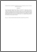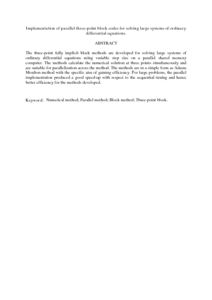# Implementation of parallel three-point block codes for solving large systems of ordinary differential equations.

## Citation

Abdul Majid, Zanariah and Suleiman, Mohamed (2010) Implementation of parallel three-point block codes for solving large systems of ordinary differential equations. International Journal of Computer Mathematics , 87 (6). pp. 1415-1429. ISSN 0020-7160

## Abstract

The three-point fully implicit block methods are developed for solving large systems of ordinary differential equations using variable step size on a parallel shared memory computer. The methods calculate the numerical solution at three points simultaneously and are suitable for parallelization across the method. The methods are in a simple form as Adams Moulton method with the specific aim of gaining efficiency. For large problems, the parallel implementation produced a good speed-up with respect to the sequential timing and hence better efficiency for the methods developed.Preview
PDF (Abstract)
Implementation of parallel three.pdfView Item